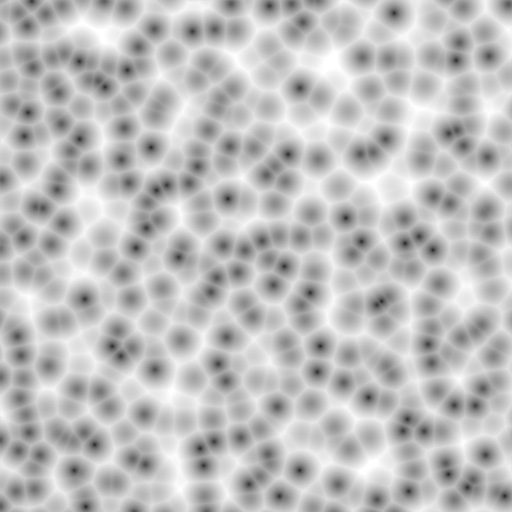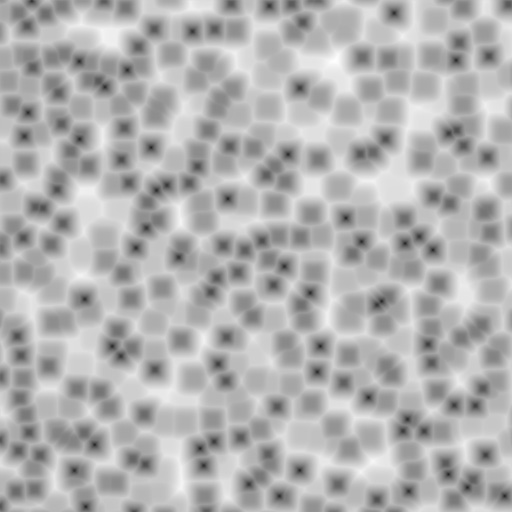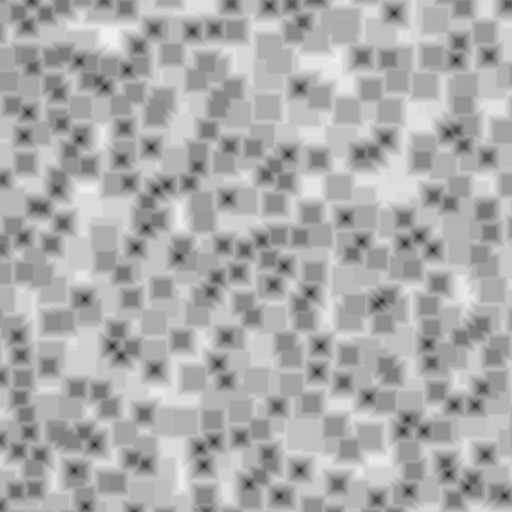Voronoi¶

The Voronoi texture is used to generate very convincing Metal, especially the “Hammered” effect. Organic shaders (e.g. scales, veins in skin).Voronoi Texture panels.

Options¶

Distance Metric

This procedural texture has seven Distance Metric options. These determine the algorithm to find the distance between cells of the texture. These options are:

• Minkowski

• Minkowski 4

• Minkowski 1/2

• Chebychev

• Manhattan

• Distance Squared

• Actual Distance

The Minkowski setting has a user definable value (the Exponent button) which determines the Minkowski exponent e of the distance function:

(xe + ye + ze)1/e

A value of one produces the Manhattan distance metric, a value less than one produces stars (at 0.5, it gives a Minkowski 1/2), and higher values produce square cells (at 4.0, it gives a Minkowski 4, at 10.0, a Chebychev). So nearly all Distance Settings are basically the same – a variation of Minkowski.

You can get irregularly-shaped rounded cells with the Actual Distance / Distance Squared options.Minkowski Exponent: 0.5 (Minkowski 1/2).¶Minkowski Exponent: 1 (Manhattan).¶Minkowski Exponent: 2 (Actual Distance).¶Minkowski Exponent: 4 (Minkowski 4).¶Minkowski Exponent: 10 (Chebychev).¶Distance Squared (more contrast than Actual Distance).¶
Feature Weights

These four sliders at the bottom of the Voronoi panel represent the values of the four Worley constants, which are used to calculate the distances between each cell in the texture based on the distance metric. Adjusting these values can have some interesting effects on the end result…

Coloring

Four settings (Intensity, Position, Position and Outline, and Position, Outline, and Intensity) that can use four different noise basis as methods to calculate color and intensity of the texture output. This gives the Voronoi texture you create with the “Worley Sliders” a completely different appearance and is the equivalent of the noise basis setting found on the other textures.